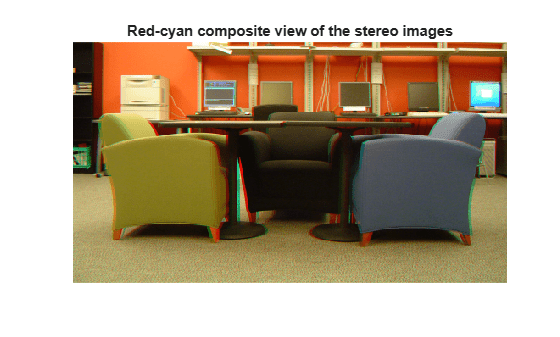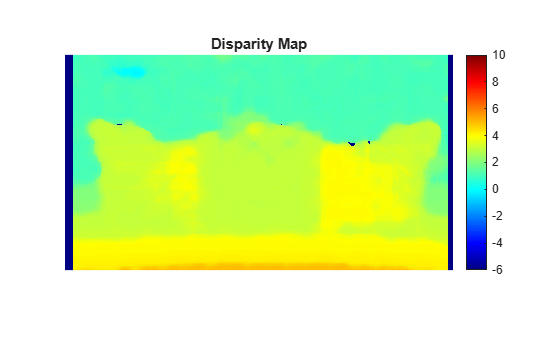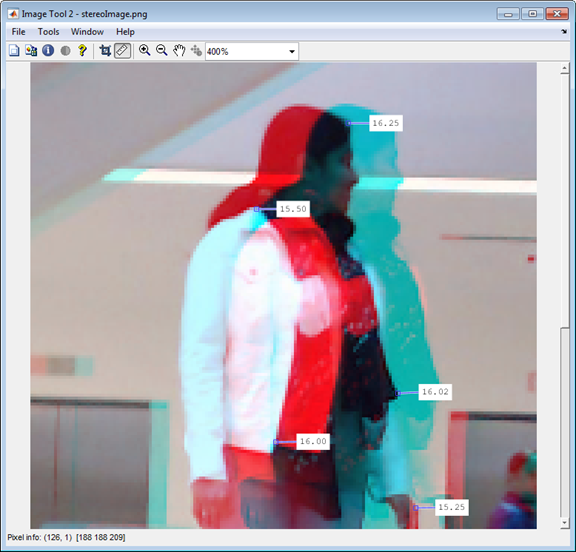disparity

(Not recommended) Disparity map between stereo images

Description

example

disparityMap = disparity(I1,I2) returns the disparity map, disparityMap, for a pair of stereo images, I1 and I2.

disparityMap = disparity(I1,I2,Name,Value) provides additional control for the disparity algorithm by using one or more Name,Value pair arguments.

Examples

collapse all

Load the images and convert them to grayscale.

Show stereo anaglyph. Use red-cyan stereo glasses to view image in 3-D.

figure
imshow(stereoAnaglyph(I1,I2));
title('Red-cyan composite view of the stereo images');Compute the disparity map.

disparityRange = [-6 10];
disparityMap = disparity(rgb2gray(I1),rgb2gray(I2),'BlockSize',...
15,'DisparityRange',disparityRange);

Display the disparity map. For better visualization, use the disparity range as the display range for imshow.

figure
imshow(disparityMap,disparityRange);
title('Disparity Map');
colormap(gca,jet)
colorbarInput Arguments

collapse all

Input image referenced as I1 corresponding to camera 1, specified in 2-D grayscale. The stereo images, I1 and I2, must be rectified such that the corresponding points are located on the same rows. You can perform this rectification with the rectifyStereoImages function.

You can improve the speed of the function by setting the class of I1 and I2 to uint8, and the number of columns to be divisible by 4. Input images I1 and I2 must be real, finite, and nonsparse. They must be the same class.

Data Types: uint8 | uint16 | int16 | single | double

Input image referenced as I2 corresponding to camera 2, specified in 2-D grayscale. The input images must be rectified such that the corresponding points are located on the same rows. You can improve the speed of the function by setting the class of I1 and I2 to uint8, and the number of columns to be divisible by 4. Input images I1 and I2 must be real, finite, and nonsparse. They must be the same class.

Data Types: uint8 | uint16 | int16 | single | double

Name-Value Arguments

Specify optional comma-separated pairs of Name,Value arguments. Name is the argument name and Value is the corresponding value. Name must appear inside quotes. You can specify several name and value pair arguments in any order as Name1,Value1,...,NameN,ValueN.

Example: 'Method','BlockMatching', specifies the 'Method' property be set to 'BlockMatching'.

Disparity estimation algorithm, specified as the comma-separated pair consisting of 'Method' and either 'BlockMatching' or 'SemiGlobal'. The disparity function implements the basic Block Matching  and the Semi-Global Block Matching  algorithms. In the 'BlockMatching' method, the function computes disparity by comparing the sum of absolute differences (SAD) of each block of pixels in the image. In the 'SemiGlobal' matching method, the function additionally forces similar disparity on neighboring blocks. This additional constraint results in a more complete disparity estimate than in the 'BlockMatching' method.

The algorithms perform these steps:

1. Compute a measure of contrast of the image by using the Sobel filter.

2. Compute the disparity for each pixel in I1.

3. Mark elements of the disparity map, disparityMap, that were not computed reliably. The function uses –realmax('single') to mark these elements.

Range of disparity, specified as the comma-separated pair consisting of 'DisparityRange' and a two-element vector. The two-element vector must be in the format [MinDisparity, MaxDisparity]. Both elements must be an integer and can be negative. MinDisparity and MaxDisparity must be in the range [-image width, image width]. The difference between MaxDisparity and MinDisparity must be divisible by 16. DisparityRange must be real, finite, and nonsparse. If the camera used to take I1 was to the right of the camera used to take I2, then MinDisparity must be negative.

The disparity range depends on the distance between the two cameras and the distance between the cameras and the object of interest. Increase the DisparityRange when the cameras are far apart or the objects are close to the cameras. To determine a reasonable disparity for your configuration, display the stereo anaglyph of the input images in the Image Viewer app and use the Distance tool to measure distances between pairs of corresponding points. Modify the MaxDisparity to correspond to the measurement.Square block size, specified as the comma-separated pair consisting of 'BlockSize' and an odd integer in the range [5,255]. This value sets the width for the square block size. The function uses the square block of pixels for comparisons between I1 and I2. BlockSize must be real, finite, and nonsparse.

Contrast threshold range, specified as the comma-separated pair consisting of 'ContrastThreshold' and a scalar value in the range (0,1]. The contrast threshold defines an acceptable range of contrast values. Increasing this parameter results in fewer pixels being marked as unreliable.ContrastThreshold must be real, finite, and nonsparse.

Minimum value of uniqueness, specified as the comma-separated pair consisting of 'UniquenessThreshold' and a nonnegative integer. Increasing this parameter results in the function marking more pixels unreliable. When the uniqueness value for a pixel is low, the disparity computed for it is less reliable. Setting the threshold to 0 disables uniqueness thresholding. UniquenessThreshold must be real, finite, and nonsparse.

The function defines uniqueness as a ratio of the optimal disparity estimation and the less optimal disparity estimation. For example:

 Let K be the best estimated disparity, and let V be the corresponding SAD (Sum of Absolute Difference) value. Consider V as the smallest SAD value over the whole disparity range, and v as the smallest SAD value over the whole disparity range, excluding K, K-1, and K+1. If v < V * (1+0.01*UniquenessThreshold), then the function marks the disparity for the pixel as unreliable.

Maximum distance for left-to-right image checking between two points, specified as the comma-separated pair consisting of 'DistanceThreshold' and a nonnegative integer. Increasing this parameter results in fewer pixels being marked as unreliable. Conversely, when you decrease the value of the distance threshold, you increase the reliability of the disparity map. You can set this parameter to an empty matrix [] to disable it. DistanceThreshold must be real, finite, and nonsparse.

The distance threshold specifies the maximum distance between a point in I1 and the same point found from I2. The function finds the distance and marks the pixel in the following way:

 Let p1 be a point in image I1. Step 1: The function searches for point p1’s best match in image I2 (left-to-right check) and finds point p2. Step 2: The function searches for p2’s best match in image I1 (right-to-left check) and finds point p3. If the search returns a distance between p1 and p3 greater than DistanceThreshold, the function marks the disparity for the point p1 as unreliable.

Minimum texture threshold, specified as the comma-separated pair consisting of 'TextureThreshold' and a scalar value in the range [0, 1). The texture threshold defines the minimum texture value for a pixel to be reliable. The lower the texture for a block of pixels, the less reliable the computed disparity is for the pixels. Increasing this parameter results in more pixels being marked as unreliable. You can set this parameter to 0 to disable it. This parameter applies only when you set Method to 'BlockMatching'.

The texture of a pixel is defined as the sum of the saturated contrast computed over the BlockSize-by-BlockSize window around the pixel. The function considers the disparity computed for the pixel unreliable and marks it, when the texture falls below the value defined by:

Texture < X* TextureThreshold * BlockSize2

X represents the maximum value supported by the class of the input images, I1 and I2.

TextureThreshold must be real, finite, and nonsparse.

Output Arguments

collapse all

Disparity map for a pair of stereo images, returned as an M-by-N 2-D grayscale image. The function returns the disparity map with the same size as the input images, I1 and I2. Each element of the output specifies the disparity for the corresponding pixel in the image references as I1. The returned disparity values are rounded to $1}{16}$th pixel.

The function computes the disparity map in three steps:

1. Compute a measure of contrast of the image by using the Sobel filter.

2. Compute the disparity for each of the pixels by using block matching and the sum of absolute differences (SAD).

3. Optionally, mark the pixels which contain unreliable disparity values. The function sets the pixel to the value returned by -realmax('single').

Tips

If your resulting disparity map looks noisy, try modifying the DisparityRange. The disparity range depends on the distance between the two cameras and the distance between the cameras and the object of interest. Increase the DisparityRange when the cameras are far apart or the objects are close to the cameras. To determine a reasonable disparity for your configuration, display the stereo anaglyph of the input images in the Image Viewer app and use the Distance tool to measure distances between pairs of corresponding points. Modify the MaxDisparity to correspond to the measurement.Compatibility Considerations

expand all

Not recommended starting in R2019a

 Konolige, K., Small Vision Systems: Hardware and Implementation, Proceedings of the 8th International Symposium in Robotic Research, pages 203-212, 1997.

 Bradski, G. and A. Kaehler, Learning OpenCV : Computer Vision with the OpenCV Library, O'Reilly, Sebastopol, CA, 2008.

 Hirschmuller, H., Accurate and Efficient Stereo Processing by Semi-Global Matching and Mutual Information, International Conference on Computer Vision and Pattern Recognition, 2005.